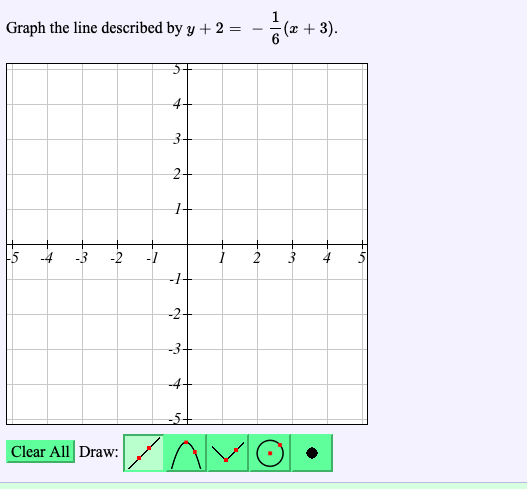# Graph the line described by y + 2(x + 3)-4Clear All Draw:

Questionhelp_outlineImage TranscriptioncloseGraph the line described by y + 2 (x + 3) -4 Clear All Draw: fullscreen
check_circleExpert Solution
Step 1

Given:

The line equation is y+2 = (-1/6)(x+3)

Step 2

Calculation:

Simplify the given equation as follows.y+2 = (-1/6)(x+3)

6y+12= (-1)(x+3)-2.

6y = -x-3-12

6y=-x-15

Obtain some coordinates of the equation as shown below.

Step 3

...

### Want to see the full answer?

See Solution

#### Want to see this answer and more?

Solutions are written by subject experts who are available 24/7. Questions are typically answered within 1 hour*

See Solution
*Response times may vary by subject and question
Tagged in

### Other